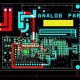# What is Feedback amplifier & its Types?

In this Tutorial we are going to learn about the feedback amplifier & its types.

Feedback Amplifier:

• When output signal return back to the input side that’s called feedback. In another words a feedback amplifier is a part or fraction of output which is combined to the input and feedback is said to exist. Thus the process of combining the fraction of output back to the input is called feedback.
• The process of combining a fraction of output energy back to input is called feedback.
• As per below circuit for simple feedback amplifier. When the loop gain AB is positive, in this circuit have two conditions which is positive or regenerative feedback.

Types of feedback amplifier: There are two types of feedback amplifier Positive feedback and Negative feedback.

Positive feedback: As per below circuit for positive circuit.

• When the feedback voltage is applied to increase the input signal that’s called positive feedback.
• When feedback voltage is applied to increase the input signal called +VE feedback, since +VE feedback cause excessive distortion and instability.
• Output is phase with i/p signal.
• I/P signal and Feedback signal gets added VIN .
• Regenerative Feedback.

Negative feedback: As per below circuit for negative circuit.

• When the feedback voltage is applied to weaken the input signal that’s called negative feedback.
• When feedback voltage is applied to weaken the input is called –VE feedback & -VE feedback reduce amplifier gain
• It has advantage such as gain stability, reduction of distortion, increase input impedance & decrease output impedance.
• Negative Feedback means input voltage reduce.
• Degenerative Amplifier.